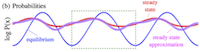# Article: Near-equilibrium measurements of nonequilibrium free energy

David A. Sivak, Gavin E. Crooks, Phys. Rev. Lett. 108, 150601 (2012)## Abstract

A central endeavor of thermodynamics is the measurement of free energy changes. Regrettably, although we can measure the free energy of a system in thermodynamic equilibrium, typically all we can say about the free energy of a non-equilibrium ensemble is that it is larger than that of the same system at equilibrium. Herein, we derive a formally exact expression for the probability distribution of a driven system, which involves path ensemble averages of the work over trajectories of the time-reversed system. From this we find a simple near-equilibrium approximation for the free energy in terms of an excess mean time-reversed work, which can be experimentally measured on real systems. With analysis and computer simulation, we demonstrate the accuracy of our approximations for several simple models.

Categories: